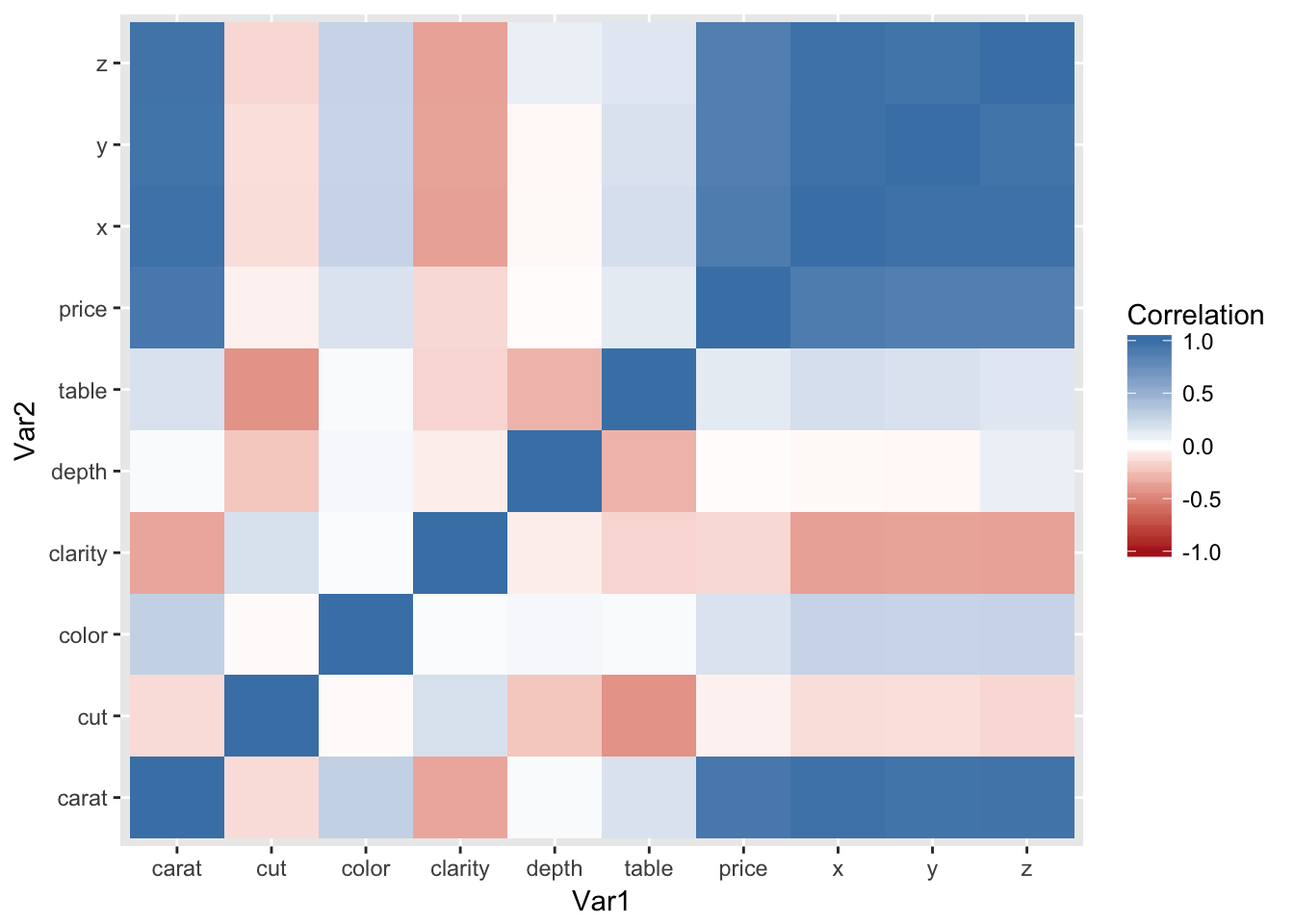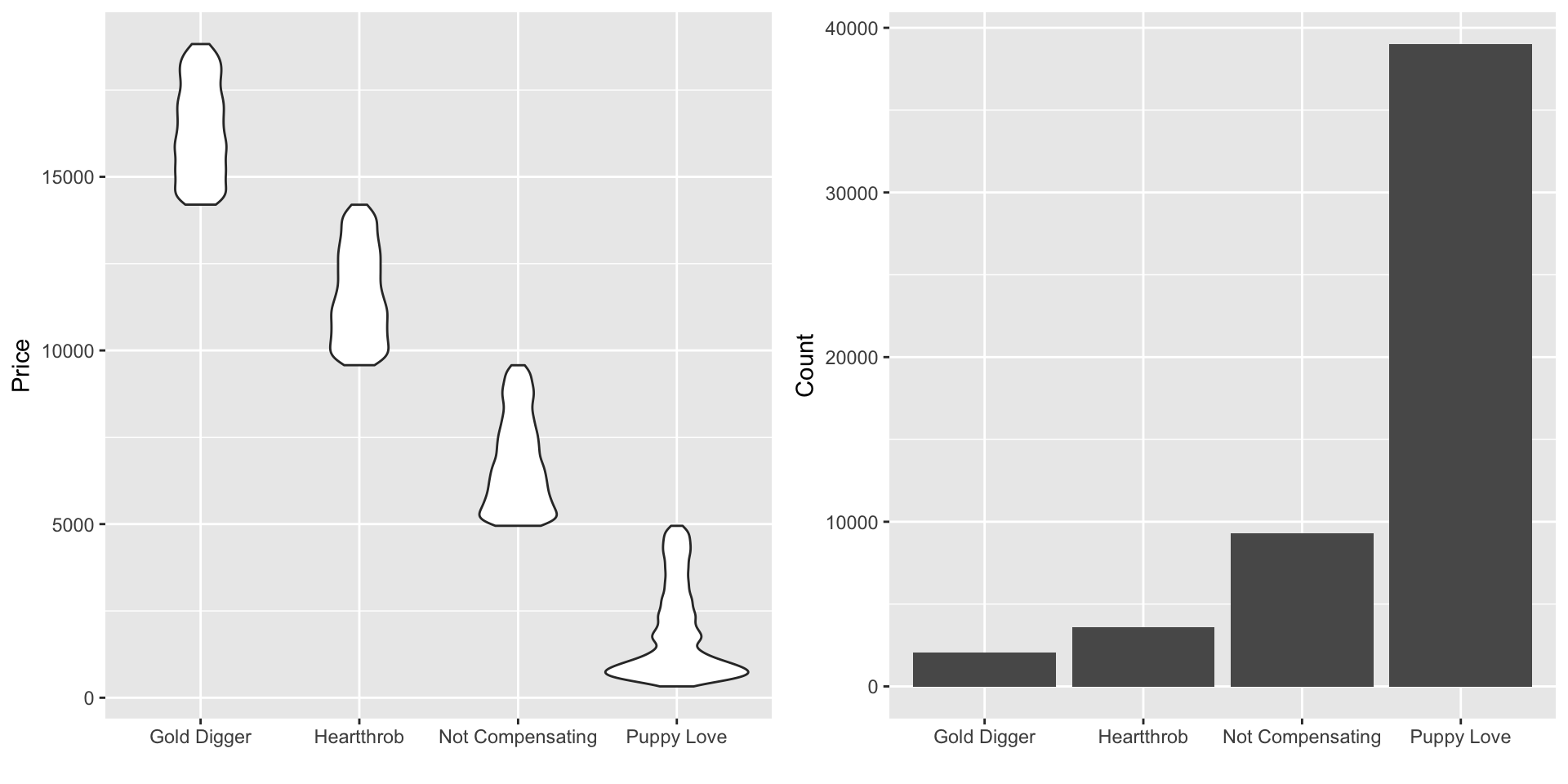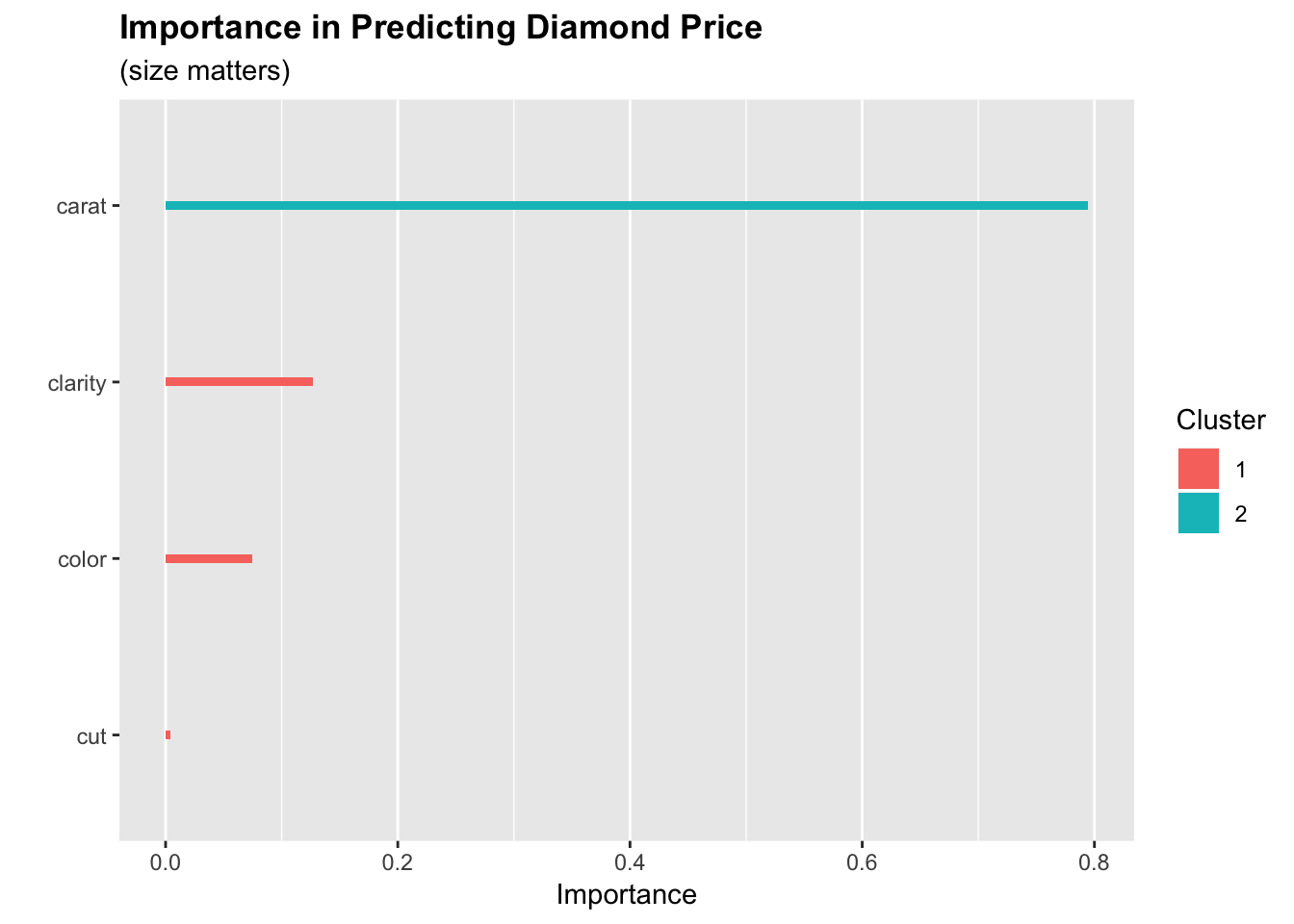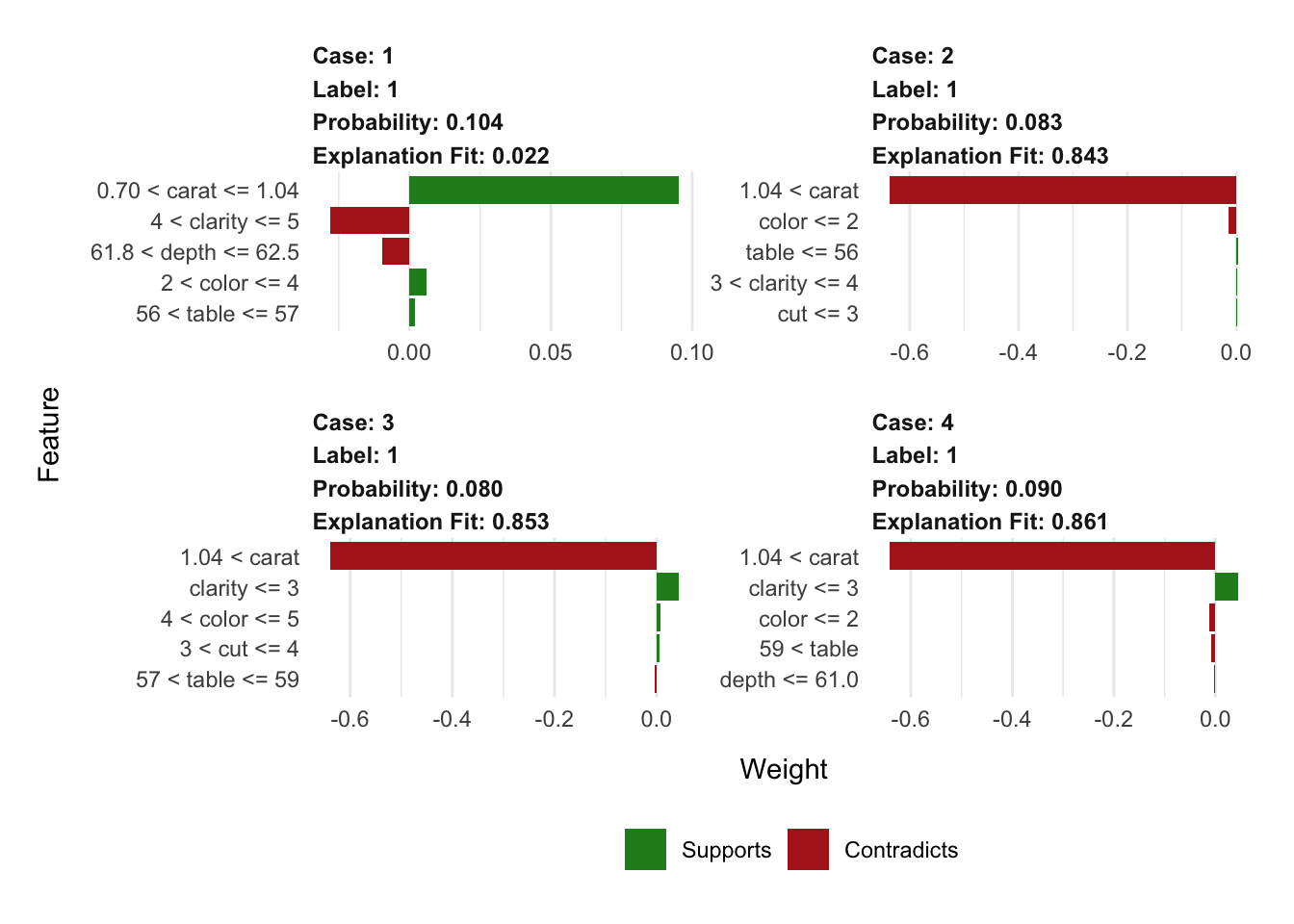Programmer/Data Scientist/Instructor・Mostly write Python & R・Big fan of OpenCV & p5js

Home

# Proposals, diamonds, xgboost, & lime

Published Feb 09, 2018

### Share

I recently got engaged! While picking out the stone for the ring I played around with the `diamonds` dataset from `ggplot2`. The analysis was around what are the main contributors to diamond pricing. (The analysis was also an excuse to play around with the `lime` package for the first time.)

To start off we’ll just load the packages that will be used in the analysis.

• dataset: The dataset that will be used is `diamonds` from `ggplot2`. To read more about the data run `?ggplot2::diamonds` in an R session.
• data manipulation: I’m a big fan of `data.table` for all data wrangling needs; it’s efficient & despite some arguments against it, I like its syntax & the flexibility it brings.
• data viz: We’ll be doing some plotting, and it’s hard to beat `ggplot2`’s output & ease of use for producing pretty static plots in R.
• analysis:
• For a quick/easy way of finding the effect diamond features have on price we’ll use `xgboost`
• Lastly, we’ll use `lime`. This package is designed to ‘explain’ classification predictions. Since it’s geared towards classification problems, we’ll bucket diamond prices into tiers; this will sacrifice some detail we could learn in feature importance, but I really wanted to play with the shiny new toy.
``````library(data.table)
library(ggplot2)
library(xgboost)
library(lime)
``````

For the first steps in the actual analysis, we’re going to convert the factors (`clarity`, `cut`, & `color`) to numeric to turn them into ranks.

Once all our data is numeric, we’ll plot a correlation heatmap to get a feel for how the features relate to one another.

``````#convert ggplot2's diamond dataset to a data.table
dt = as.data.table(diamonds)

#convert all factors in dt to numeric
dt_factors = names(which(vapply(dt, is.factor, logical(1))))
dt[, (dt_factors) := lapply(.SD, as.numeric), .SDcols=dt_factors]

#plot correlations in data
ggplot(data = melt(cor(dt)), aes(x=Var1, y=Var2, fill=value)) +
geom_tile() +
high = "steelblue",
limit = c(-1,1),
name="Correlation")
``````Not too big of a surprise that the physical dimensions of the diamond (`x`, `y`, & `z`) are highly correlated to `caret`. These will be dropped for the remainder of the analysis.

As mentioned before, one reason for this post is to have an excuse to try out the `lime` package. The `lime` package is used to explain classification models’ predictions. So to use the package, we’ll have to change this to a classification problem; the prices will be binned into 4 categories using `cut`.

The below chunk does the binning and produces some plots to summarize each bin.

``````#drop x, y, z
dt[, (c("x","y","z")) := NULL]

#bin price (zero indexed for xgboost)
dt[, y := cut(price, 4, labels = FALSE) - 1]

#dictionary of bin labels
label_dict = c("Puppy Love", "Not Compensating",
"Heartthrob", "Gold Digger")
names(label_dict) = as.character(0:3)

#group all y info into single dt for easy plotting
y_dt = data.table(Price=dt\$price,
num_label=dt\$y,
#look up label in dictionary
label=label_dict[as.character(dt\$y)])

#price distribution by bin
ggplot(y_dt, aes(label, Price)) +
geom_violin() +
labs(x="")

#observation count by bin
ggplot(y_dt, aes(label)) +
geom_bar() +
labs(x="", y="Count")
``````We’re now ready to get a better grasp on feature importance. An easy way to get a feel for the importance is to use an `xgboost` model. There are probably good arguments to use different models in this specific case, but I’ve grown to like `xgboost` for this kind of exploration due to its flexibility across the target data type.

``````#convert  x&y to type xgboost is expecting
x = as.matrix(dt[,-c("price", "y")])
y = dt\$y

#won't fuss too much over the params
#since this is for exploration instead of prediction
n_rounds  = 50
xg_params = list(max_depth=5,
eta=.03,
subsample=.7,
colsample_bytree=.7,
objective = "multi:softprob",
eval_metric = "mlogloss",
num_class = 4)

#train model
set.seed(42)
xgb_mod = xgboost(x, y,
params = xg_params,
nrounds = n_rounds,
verbose = 0)

#extract importance in prediction
importance_dt = xgb.importance(colnames(x), xgb_mod)

#plot importance
xgb.ggplot.importance(importance_dt) +
labs(title="Importance in Predicting Diamond Price",
subtitle="(size matters)",
x="")
``````Only 4 of the diamond features were important enough to be included in the model, and we can see that `carat` carries most of the influence on price by itself.

The last part of the analysis will be focused on using the `lime` package. In the below chunk we create an `explainer` by providing our data and `xgboost` model. Then we sample 1 observation from each of our 4 classes to be explained.

``````#make lime diamond class explainer
explainer = lime(dt[,-c("price", "y")], xgb_mod)

#get observation from each class
set.seed(8675309)
rand_diamonds = dt[, lapply(.SD, function(i) sample(i, 1)), by=y]

#inspect
print(rand_diamonds)
``````Lets tell the explainer that each of these 4 instances is in the cheapest class of diamonds. We do this by specifying `1` in the `label` argument (yeah, I know this conflicts with the `0` label that `xgboost` is actually using).

The explainer will then tell us what evidence supports and contradicts this assigned label of `1`. We can see that only the diamond plotted in the top left has legimate evidence to classify it as the cheapest class; this is for good reason since it is the only observation labeled correctly here.

An interesting aspect of these plots compared to the `xgboost` importance output, is that they incorporate more of the features in the explanation than were actually used in the model for prediction.

``````#drop y vars
rand_diamonds = rand_diamonds[,-c("price", "y")]

#explain why each diamond is or isn't in the cheapest class
#with support from 5 features
explanation = explain(rand_diamonds, explainer,
label = 1, n_features = 5)

#viz explanations
plot_features(explanation)
``````### Post Analysis Cat Pic

If you’ve made it this far (or clicked the link in the intro), have a look at my kitty Jasper helping to announce the good news!